# Bürmann-Lagrange series

(Redirected from Bürmann–Lagrange series)

Lagrange series

A power series which offers a complete solution to the problem of local inversion of holomorphic functions. In fact, let a function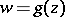of the complex variablebe regular in a neighbourhood of the point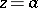, and let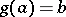and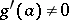. Then there exists a regular functionin some neighbourhood of the point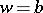of the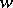-plane which is the inverse to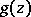and is such that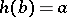. Moreover, ifis any regular function in a neighbourhood of the point, then the composite functioncan be expanded in a Bürmann–Lagrange series in a neighbourhood of the point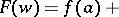(*)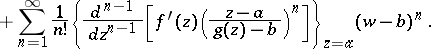The inverse of the functionis obtained by setting.

The expansion (*) follows from Bürmann's theorem : Under the assumptions made above on the holomorphic functionsand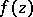, the latter function may be represented in a certain domain in the-plane containingin the form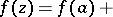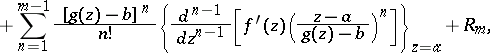where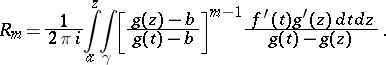Hereis a contour in the-plane which encloses the points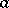and, and is such that if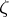is any point inside, then the equationhas no roots onor insideother than the simple root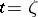.

The expansion (*) for the case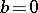was obtained by J.L. Lagrange .

If the derivative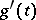has a zero of orderat the point, there is the following generalization of the Bürmann–Lagrange series for the multi-valued inverse function :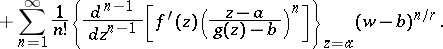Another generalization (see, for example, ) refers to functionsregular in an annulus; instead of the series (*), one obtains a series with positive and negative powers of the difference.